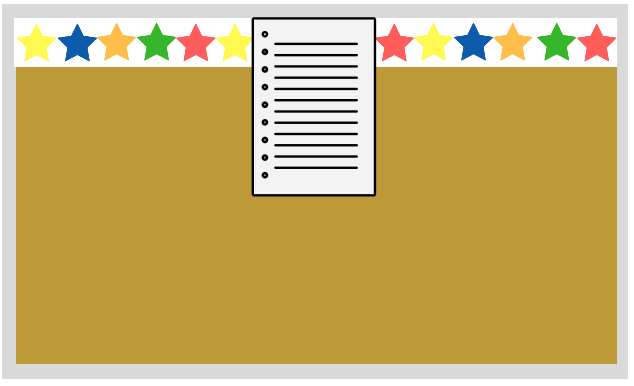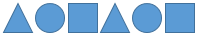# Multi-Digit Division

## Objective

Identify and extend repeating shape patterns.

## Common Core Standards

### Core Standards

?

• 4.OA.C.5 — Generate a number or shape pattern that follows a given rule. Identify apparent features of the pattern that were not explicit in the rule itself. For example, given the rule "Add 3" and the starting number 1, generate terms in the resulting sequence and observe that the terms appear to alternate between odd and even numbers. Explain informally why the numbers will continue to alternate in this way.

?

• 3.OA.D.9

## Criteria for Success

?

1. Identify the rule of a repeating shape pattern and use letters to describe it (e.g., an ABB pattern) (MP.7, MP.8).
2. Use the rule of a repeating shape pattern to extend it to subsequent terms (MP.8).
3. Use the rule of a repeating shape pattern to find its ${n^{\mathrm{th}}}$ term (MP.8).
4. Use the rule of a repeating shape pattern to identify missing terms (MP.8).

## Tips for Teachers

?

“Properties of repeating patterns of shapes can be explored with division. For example, to determine the 100th shape in a pattern that consists of repetitions of the sequence “square, circle, triangle,” the fact that when we divide 100 by 3 the whole number quotient is 33 with remainder 1 tell us that after 33 full repeats, the 99th shape will be a triangle (the last shape in the repeating pattern), so the 100th shape is the first shape in the pattern, which is a square” (OA Progression, p. 31). Thus, Lesson 16’s additional cluster content of repeating shape patterns connects across clusters to the major work of interpreting remainders (4.OA.3) as well as across domains to the major work of multi-digit division (4.NBT.6).

#### Remote Learning Guidance

If you need to adapt or shorten this lesson for remote learning, we suggest prioritizing Anchor Task 2 (benefits from worked example). Find more guidance on adapting our math curriculum for remote learning here.

#### Fishtank Plus

• Problem Set
• Student Handout Editor
• Vocabulary Package

?

### Problem 1

Ms. Roth used a patterned border around her bulletin board. She then posted some student work on her bulletin board. This is what it looks like:What part of the border did Ms. Roth cover up?

### Problem 2

A pattern is shown below.1. What will be the next shape in the pattern?
2. What will be the 20th shape in the pattern The 50th shape in the pattern?

## Problem Set & Homework

#### Discussion of Problem Set

• Look at #1. How did you figure out what the 75th shape would be?
• Look at #3. How did you figure out what the missing shapes were?
• Look at #6. What were some patterns you came up with?

?

Belinda made the following shape pattern.What will be the ${100^{\mathrm{th}}}$ term in the pattern? Show or explain how you know.

### Mastery Response

?